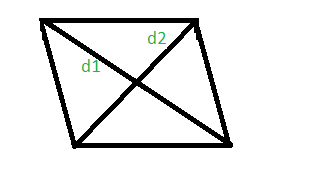# Program to calculate area and perimeter of a rhombus whose diagonals are given

Given the length of diagonals of a rhombus, d1 and d2. The task is to find the perimeter and the area of that rhombus.
A rhombus is a polygon having 4 equal sides in which both the opposite sides are parallel, and opposite angles are equal.Examples:

Input: d1 = 2 and d2 = 4
Output: The area of rhombus with diagonals 2 and 4 is 4.
The perimeter of rhombus with diagonals 2 and 4 is 8.

Input: d1 = 100 and d2 = 500
Output: The area of rhombus with diagonals 100 and 500 is 25000.
The perimeter of rhombus with diagonals 100 and 500 is 1019.


## Recommended: Please try your approach on {IDE} first, before moving on to the solution.

Area of the rhombus =Perimeterof the rhombus =Below is the implementation of above approach:

## C++

 // C++ Program to calculate area and perimeter  // of a rhombus using diagonals  #include  #include  using namespace std;     // calculate area and perimeter of a rhombus  int rhombusAreaPeri(int d1, int d2)  {      long long int area, perimeter;         area = (d1 * d2) / 2;      perimeter = 2 * sqrt(pow(d1, 2) + pow(d2, 2));         cout << "The area of rhombus with diagonals "           << d1 << " and " << d2 << " is " << area << "." << endl;         cout << "The perimeter of rhombus with diagonals "           << d1 << " and " << d2 << " is " << perimeter << "." << endl;  }     // Driver code  int main()  {         int d1 = 2, d2 = 4;      rhombusAreaPeri(d1, d2);         return 0;  }

## Java

 // Java program to calculate area and perimeter  // of a rhombus using diagonals  import java.io.*;     class GFG {       // calculate area and perimeter of a rhombus  static int rhombusAreaPeri(int d1, int d2)  {       int area, perimeter;         area = (d1 * d2) / 2;      perimeter = (int)(2 * Math.sqrt(Math.pow(d1, 2) + Math.pow(d2, 2)));         System.out.println( "The area of rhombus with diagonals "         + d1 + " and " + d2 + " is " + area + ".");         System.out.println("The perimeter of rhombus with diagonals "         +d1 + " and " + d2 + " is " + perimeter + ".");          return 0;  }     // Driver code            public static void main (String[] args) {          int d1 = 2, d2 = 4;      rhombusAreaPeri(d1, d2);      }  }  // This code is contributed by anuj_67..

## Python3

 # Python 3 Program to calculate   # area and perimeter of a rhombus  # using diagonals  from math import sqrt, pow    # calculate area and perimeter  # of a rhombus  def rhombusAreaPeri(d1, d2):      area = (d1 * d2) / 2     perimeter = 2 * sqrt(pow(d1, 2) +                           pow(d2, 2))         print("The area of rhombus with diagonals",                   d1, "and", d2, "is", area, ".")         print("The perimeter of rhombus with diagonals",                  d1, "and", d2, "is", perimeter, "." )     # Driver code  if __name__ == '__main__':      d1 = 2     d2 = 4     rhombusAreaPeri(d1, d2)     # This code is contributed   # by Surendra_Gangwar

## C#

 // C# program to calculate area   // and perimeter of a rhombus   // using diagonals  using System;     class GFG   {     // calculate area and perimeter   // of a rhombus  static int rhombusAreaPeri(int d1,                               int d2)  {      int area, perimeter;         area = (d1 * d2) / 2;      perimeter = (int)(2 * Math.Sqrt(Math.Pow(d1, 2) +                                       Math.Pow(d2, 2)));         Console.WriteLine( "The area of rhombus with " +                          "diagonals " + d1 + " and " +                             d2 + " is " + area + ".");         Console.WriteLine("The perimeter of rhombus " +                         "with diagonals " + d1 + " and " +                           d2 + " is " + perimeter + ".");          return 0;  }     // Driver code  public static void Main ()   {      int d1 = 2, d2 = 4;      rhombusAreaPeri(d1, d2);  }  }     // This code is contributed by anuj_67..

## PHP

 

Output:

The area of rhombus with diagonals 2 and 4 is 4.
The perimeter of rhombus with diagonals 2 and 4 is 8.


Attention reader! Don’t stop learning now. Get hold of all the important DSA concepts with the DSA Self Paced Course at a student-friendly price and become industry ready.

My Personal Notes arrow_drop_upCheck out this Author's contributed articles.

If you like GeeksforGeeks and would like to contribute, you can also write an article using contribute.geeksforgeeks.org or mail your article to contribute@geeksforgeeks.org. See your article appearing on the GeeksforGeeks main page and help other Geeks.

Please Improve this article if you find anything incorrect by clicking on the "Improve Article" button below.